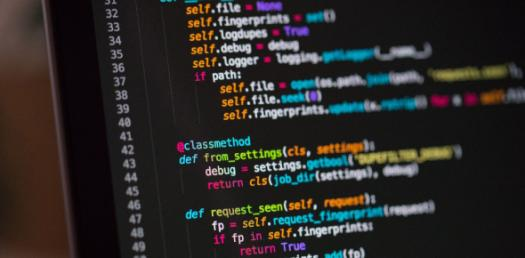# Trivia Questions Quiz On Integers, Floating-point Numbers, And Characters!

26 Questions | Total Attempts: 42SettingsWelcome to the Trivia Questions Quiz on Integers, Floating-point Numbers, and Characters! It is perfect for every student having a hard time when it comes to solving math problems that have these characteristic problems. Do take it up and get to see if you might need to take extra quizzes. All the best, and keep practicing until you perfect your skills. All the best!

• 1.
A linked list is a data structure that combines a set of related data and the methods that manipulate that data.
• A.

True

• B.

False

• 2.
Two&#8217;s complement notation encodes a real number as a mantissa multiplied by a power (exponent) of two.
• A.

True

• B.

False

• 3.
What coding format encodes a real number as a mantissa multiplied by a power (exponent) of two?
• A.

Two’s complement

• B.

Floating point

• 4.
The result of adding, subtracting, or multiplying two integers may result in overflow, but never underflow or truncation.
• A.

True

• B.

False

• 5.
Commonly used standards for floating-point representation are defined by the ____.
• A.

IEEE

• B.

ANSI

• 6.
Which of the following character coding schemes include control codes for I/O devices?
• A.

EBCDIC

• B.

ASCII

• 7.
What data type is an array of data items, each of which contains a key value and a pointer to another data item?
• A.

• B.

Index

• 8.
Which data encoding method is most similar to scientific notation?
• A.

Floating point notation

• B.

Two’s complement notation

• 9.
What data structure is normally used to store a string?
• A.

Array

• B.

Record

• 10.
The term ____ describes the number of bits used to represent a numeric value.
• A.

Range

• B.

Compactness

• 11.
A(n) data structure is a data item composed of multiple primitive data items.
• A.

True

• B.

False

• 12.
A sequence of characters that forms a meaningful word, phrase, or other user group is a(n) string.
• A.

True

• B.

False

• 13.
Which of the following is not considered one of the five primitive data types?
• A.

• B.

• 14.
In EBCDIC character coding, the character &#039;1&#039; and the integer 1 are represented by identical bit strings.
• A.

True

• B.

False

• 15.
Which of the following character coding schemes include control codes for I/O devices?
• A.

ASCII

• B.

BCD

• C.

EBCDIC

• D.

IEEE

• 16.
Underflow occurs when a negative exponent is too large in absolute value to fit within the bits allocated to store it.
• A.

True

• B.

False

• 17.
In EBCDIC character coding, the character &#039;1&#039; and the integer 1 are represented by identical bit strings.
• A.

True

• B.

False

• 18.
How many bits are used to represent a character under the ASCII coding standard?
• A.

7

• B.

8

• 19.
The assignment of numeric codes to characters follows a specific order called a(n) ____.
• A.

Record Separator

• B.

Collating Sequence

• 20.
What coding format encodes a real number as a mantissa multiplied by a power (exponent) of two?
• A.

Two’s complement

• B.

Floating point

• 21.
Which of the following does not result from floating-point math operations?
• A.

Underflow

• B.

Overflow

• C.

Truncation

• D.

Two’s complement

• 22.
The inflection point is the bit of lowest magnitude within a byte or bit string.
• A.

True

• B.

False

• 23.
A(n) index is an array of data items, each of which contains a key value and a pointer to another data item.
• A.

True

• B.

False

• 24.
Two&#8217;s complement notation represents positive values as ordinary binary numbers.
• A.

True

• B.

False

• 25.
What does the 0x in the written text 0x1010 signify?
• A.

1010 is a binary number

• B.

1010 is a two’s complement value

• C.Back to top